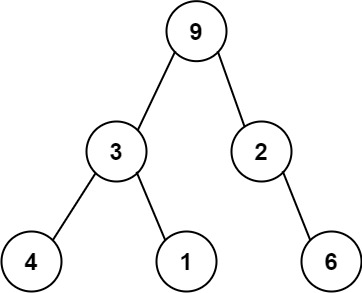331. Verify Preorder Serialization of a Binary Tree
Medium
2.1K
107

One way to serialize a binary tree is to use preorder traversal. When we encounter a non-null node, we record the node's value. If it is a null node, we record using a sentinel value such as `'#'`.For example, the above binary tree can be serialized to the string `"9,3,4,#,#,1,#,#,2,#,6,#,#"`, where `'#'` represents a null node.

Given a string of comma-separated values `preorder`, return `true` if it is a correct preorder traversal serialization of a binary tree.

It is guaranteed that each comma-separated value in the string must be either an integer or a character `'#'` representing null pointer.

You may assume that the input format is always valid.

• For example, it could never contain two consecutive commas, such as `"1,,3"`.

Note: You are not allowed to reconstruct the tree.

Example 1:

```Input: preorder = "9,3,4,#,#,1,#,#,2,#,6,#,#"
Output: true
```

Example 2:

```Input: preorder = "1,#"
Output: false
```

Example 3:

```Input: preorder = "9,#,#,1"
Output: false
```

Constraints:

• `1 <= preorder.length <= 104`
• `preorder` consist of integers in the range `[0, 100]` and `'#'` separated by commas `','`.
Accepted
129.6K
Submissions
290.3K
Acceptance Rate
44.7%

Seen this question in a real interview before?
1/4
Yes
No

Discussion (0)

Related Topics